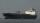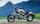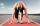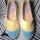# Sprinter

Sprinter runs the relay 4 x 400 m to the handover at speed 42 km/h. A second runner is at the start of the handover area 20 m long and runs when it is the first sprinter at distance 10 m. Calculate the speed at which the second runner must run in order to handover occurred at the end of the handover area. Consider that the speed of both runners are constant.

Result

v =  28 km/h

#### Solution:

v/0.020 = 42/(0.020+0.010)

50v = 1400

v = 28

Calculated by our simple equation calculator.

Leave us a comment of example and its solution (i.e. if it is still somewhat unclear...):

Showing 0 comments:Be the first to comment!#### To solve this example are needed these knowledge from mathematics:

Do you have a linear equation or system of equations and looking for its solution? Or do you have quadratic equation?

## Next similar examples:

1. The shipThe ship went upstream speed of 20 km/h in relation to water. River flows at speed 10 km/h. After half an hour, he stopped and returned downstream to the starting point. How long it took way back when even if downstream speed of ship is 20 km/h in relation
2. Bus and MilanThe bus started from the village at speed 60 km/h. After one kilometer, when Milan came to bus stop on a motorcycle with grandmother. When they found that bus drove away he try to catch it. At the next stop, a distance of 3km caught up bus. How quickly had
3. Runner 2Marian run 12 meters in 8 seconds. How far would Marian run for 70 seconds, if still run at the same pace?
4. Cenda and PepaCenda and Pepa went to the event. Cenda started alone. Canda started after him for 20 minutes. How long took Cenda to catch him? Cenda traveling at 15 km/h, and Pepa traveling at 25 km/h.
5. Cars 6At 9:00 am two cars started from the same town and traveled at a rate of 35 miles per hour and the other car traveled at a rate of 40 miles per hour. After how many hours will the cars be 30 miles apart?
6. BicycleThe bicycle pedal gear has 36 teeth, the rear gear wheel has 10 teeth. How many times turns rear wheel, when pedal wheel turns 120x?
7. DonutsFind how many donuts each student will receive if you share 126 donuts in a ratio of 1:5:8
8. Round-tripA woman works at a law firm in city A which is about 50 miles from city B. She must go to the law library in city B to get a document. Find how long it takes her to drive​ round-trip if she averages 40 mph.
9. Liters od milkThe cylinder-shaped container contains 80 liters of milk. Milk level is 45 cm. How much milk will in the container, if level raise to height 72 cm?
10. Masons1 mason casts 30.8 meters square in 8 hours. How long casts 4 masons 178 meters square?
11. Simple equationSolve for x: 3(x + 2) = x - 18
12. StormSo far, a storm has traveled 35 miles in 1/2 hour in direction straight to observer. If it is currently 5:00 p. m. and the storm is 105 miles away from you, at what time will the storm reach you? Explain how you solved the problem.
13. 1.5 divided1.5 divided by 1 = w divided by 4
14. CandyPeter had a sachet of candy. He wanted to share with his friends. If he gave them 30 candies, he would have 62 candies. If he gave them 40 candies, he would miss 8 candies. How many friends did Peter have?
15. Fifth of the numberThe fifth of the number is by 24 less than that number. What is the number?
16. Six yearsIn six years Jan will be twice as old as he was six years ago. How old is he?
17. Unknown numberIdentify unknown number which 1/5 is 40 greater than one tenth of that number.